## A submarine is 2.84 102 m horizontally from shore and 1.00 102 m beneath the surface of the water. A laser beam is sent from the submarine s

Question

A submarine is 2.84 102 m horizontally from shore and 1.00 102 m beneath the surface of the water. A laser beam is sent from the submarine so that the beam strikes the surface of the water 2.34 102 m from the shore. A building stands on the shore, and the laser beam hits a target at the top of the building. The goal is to find the height of the target above sea level.

in progress 0
7 months 2021-07-20T02:48:34+00:00 1 Answers 6 views 0

468 m

Explanation:

So the building and the point where the laser hit the water surface make a right triangle. Let’s call this triangle ABC where A is at the base of the building, B is at the top of the building, and C is where the laser hits the water surface. Similarly, the submarine, the projected submarine on the surface and the point where the laser hit the surface makes a another right triangle CDE. Let D be the submarine and E is the other point.

The length CE is length AE – length AC = 284 – 234 = 50 m

We can calculate the angle ECD: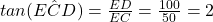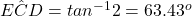This is also the angle ACB, so we can find the length AB: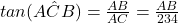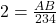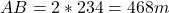So the height of the building is 468m